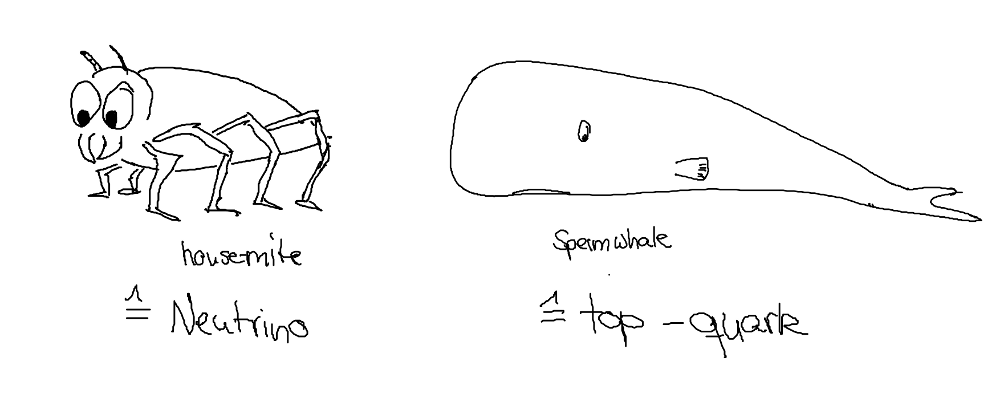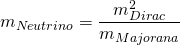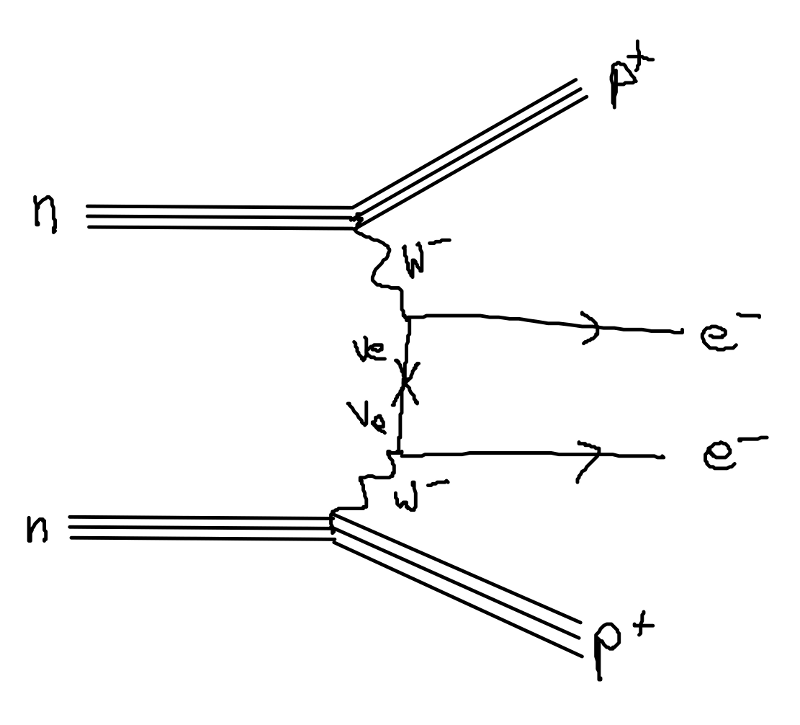Categories
Pages
-## Species

August 9th, 2014 | by

The origin and size of the neutrinos masses is one of the unsolved mysteries in High energy physics. Their masses must be very small (< 1eV) in comparison to the other elementary particles: if the neutrino had the weight of a house mite, the top quark would have the mass of a sperm whale! For theorists, this hierarchy in the masses is really unsatisfying, and the question is if there is a mechanism that makes the neutrino mass automatically that small.New results from a neutrino experiment, EXO 200, were published in Nature in June. This and other experiments like GERDA, Moon, KamLAND-Zen, DCBA, CUORE and COBRA try to find out more about the nature of the neutrinos which could explain the mass hierarchy. Neutrinos are fermions, but there are two species of fermions: Majorana and Dirac fermions. While all quarks and charged leptons are Dirac fermions, it is not clear whether Neutrinos are Majorana or Dirac fermions.
So what is the big difference?
One important difference is that Majorana fermions are their own antiparticles, while Dirac fermions can’t be, implying that the charged fermions such as electrons have to be Dirac fermions. Neutrinos are neutral, so they can be Majorana fermions. The type of fermion has influence on how one can write down mass terms in the equation of motion for this particle.
Every fermion has four components (you could call them degrees of freedom), which can be grouped into two components representing the particle and two representing the antiparticle. To build a Majorana fermion, you choose one component of the particle and one of the antiparticle. Due to this connection, the particle is its own antiparticle. To build a Dirac fermion, you leave them in fermion and antifermion groups (and thus have charged fermions).

This has implications for the mass term in the equation of motion of the fermion: The Dirac mass term connects the two components of the particle (or the antiparticle). The Majorana mass term however, connects the two components of the particle and the antiparticle. To make it even more confusing, you can write down both mass terms for Majorana particles since they are neutral.

This is pretty heavy stuff. But bottom line is, there are two kind of mass terms, and two kind of fermions. So how does that help with the mass hierarchy?

Basically, one writes down a Dirac mass term that is small, and a Majorana mass term that is large. After some math, which you have to perform in order to get the physical neutrino masses, one ends up with a nice correlation:
The physical masses depend on the Dirac and Majorana masses such that, if you make the Majorana mass very very heavy, the physical mass of the neutrino becomes very very tiny:This is exactly what one is interested in: a mechanism, that automatically drives the neutrino mass to a very small value without any magical contribution. Since this behaviour gives the impression of a see-saw to physicists, it is called the See-Saw mechanism. Let’s put in some numbers: if we assume the Dirac mass of the order of the Top-quark (as the largest mass we know) then we need a heavy scale of the order of 10^14 GeV for the Majorana mass to get a neutrino with the mass <1 eV.
We should not forget to mention here, that this mechanism includes a new, unobserved neutrino, which automatically becomes very heavy due to this mechanism.

So, you might ask, that is a nice idea, but is it actually realized in nature?

That is what EXO 200 and the other experiments actually try to measure via the neutrinoless double beta decay. Let us first shed some light on the normal double beta decay. This is the same as the common beta decay (the decay of a neutron into a electron, a proton and a neutrino), but just two of them in one nucleus at the same time (for some atoms, the single neutrino decay is energetically forbidden). The end products are two neutrinos and two electrons. So what is the neutrinoless double beta decay?

If neutrinos are Majorana particles, they are their own antiparticles. This implies that they can annihilate with each other. This means, if  two neutrons in a nuclei decay at the same time, then there is a small chance, that the two neutrinos annihilate with each other! Then, the only decay products are electrons and the energy of the two electrons is exactly the energy of the decay.In the EXO 200 experiment, one looks for these decays, and if it is not  measured, one can derive a limit on the probability of this process. This was done, and the experiment could put a limit on the half-life of this decay with 1.1* 10^{25} years. From this limit, one can also calculate an upper limit for the neutrino mass for specific realisations of the see-saw mechanism. The limits from GERDA experiment  “phase I”  are comparable with 2.1 * 10^25 years. The limits are supposed to improve by a factor of 10 in the next years.